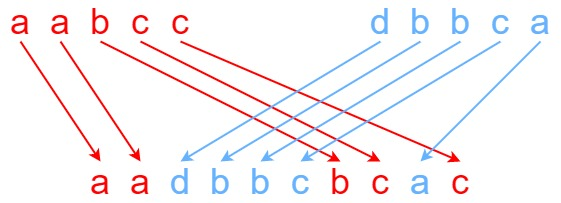### LeetCode• ㊗️
• 大家
• offer
• 多多！

## Problem

Given strings s1, s2, and s3, find whether s3 is formed by an interleaving of s1 and s2.

An interleaving of two strings s and t is a configuration where s and t are divided into n and m substrings respectively, such that:

• $s = s_1 + s_2 + … + s_n$
• $t = t_1 + t_2 + … + t_m$
• $|n - m| <= 1$
• The interleaving is $s_1 + t_1 + s_2 + t_2 + s_3 + t_3 + …$ or $t_1 + s_1 + t_2 + s_2 + t_3 + s_3 + …$

Note: a + b is the concatenation of strings a and b.

Example 1:Input: s1 = "aabcc", s2 = "dbbca", s3 = "aadbbcbcac"
Output: true
Explanation: One way to obtain s3 is:
Split s1 into s1 = "aa" + "bc" + "c", and s2 into s2 = "dbbc" + "a".
Interleaving the two splits, we get "aa" + "dbbc" + "bc" + "a" + "c" = "aadbbcbcac".
Since s3 can be obtained by interleaving s1 and s2, we return true.


Example 2:

Input: s1 = "aabcc", s2 = "dbbca", s3 = "aadbbbaccc"
Output: false
Explanation: Notice how it is impossible to interleave s2 with any other string to obtain s3.


Example 3:

Input: s1 = "", s2 = "", s3 = ""
Output: true


Constraints:

• $0 <= s1.length, s2.length <= 100$
• $0 <= s3.length <= 200$
• s1, s2, and s3 consist of lowercase English letters.

Follow up: Could you solve it using only $O(s2.length)$ additional memory space?

## Code

s1 = “aabcc”, s2 = “dbbca”, s3 = “aadbbcbcac”

d b b c a
0 1 2 3 4 5
0 T F F F F F
a 1 T F F F F F
a 2 T T T T T F
b 3 F T T F T F
c 4 F F T T T T
c 5 F F F T F T
class Solution {
public boolean isInterleave(String s1, String s2, String s3) {
int len1 = s1.length();
int len2 = s2.length();
int len3 = s3.length();

if(len1 + len2 != len3) return false;
if(len1 == 0 && len2 == 0 && len3 == 0) return true;

boolean [][] dp = new boolean[len1 + 1][len2 + 1];
dp = true;

for (int j = 1; j <= len2; j++) {
if(s2.charAt(j - 1) == s3.charAt(j - 1) && dp[j - 1] == true) {
dp[j] = true;
}
}

for(int i = 1; i <= len1; i++) {
if(s1.charAt(i - 1) == s3.charAt(i - 1) && dp[i - 1] == true) {
dp[i] = true;
}
}

for(int i = 1; i <= len1; i++) {
for(int j = 1; j <= len2; j++) {
if(s1.charAt(i - 1) == s3.charAt(i + j - 1) && dp[i - 1][j] == true) {
dp[i][j] = true;
}

if(s2.charAt(j - 1) == s3.charAt(i + j - 1) && dp[i][j - 1] == true) {
dp[i][j] = true;
}
}
}

return dp[len1][len2];
}
}